# Schwarzschild metric facts for kids

Kids Encyclopedia Facts

The Schwarzschild metric was calculated by Karl Schwarzschild as a solution to Einstein's field equations in 1916. Also known as Schwarzschild solution, it is an equation from general relativity in the field of astrophysics. A metric refers to an equation which describes spacetime; in particular, a Schwarzschild metric describes the gravitational field around a Schwarzschild black hole - a non-rotating, spherical black hole with no magnetic field, and where the cosmological constant is zero.

It is essentially an equation that describes how a particle moves through the space near a black hole.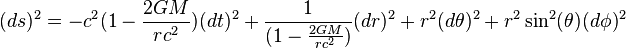$(ds)^{2}=-c^{2}(1-{\frac {2GM}{rc^{2}}})(dt)^{2}+{\frac {1}{(1-{\frac {2GM}{rc^{2}}})}}(dr)^{2}+r^{2}(d\theta )^{2}+r^{2}\sin ^{2}(\theta)(d\phi)^{2}$

## Derivation

Although a more complicated way of calculating the Schwarzschild metric can be found using Christoffel Symbols, it can also be derived using the equations for escape velocity (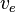$v_{e}$), time dilation (dt'), length contraction (dr'):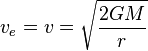$v_{e} = v = \sqrt{\frac{2GM}{r}}$ (1)

v is the velocity of the particle
G is the gravitational constant
M is the mass of the black hole
r is how close the particle is to the heavy object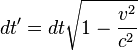$dt'=dt \sqrt{1-\frac{v^{2}}{c^{2}}}$ (2)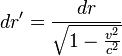$dr'=\frac{dr}{\sqrt{1-\frac{v^{2}}{c^{2}}}}$ (3)

dt' is the particle's true change in time
dt is the particle's change in time
dr' is the true distance traveled
dr is the particle's change in distance
v is the velocity of the particle
c is the speed of light

Note: the true time interval and true distance traveled by the particle are different than the time and distance calculated in classical physics calculations, since it is traveling in such a heavy gravitational field!

Using the equation for flat spacetime in spherical coordinates: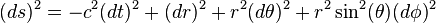$(ds)^{2}=-c^{2}(dt)^{2}+(dr)^{2}+r^{2}(d\theta )^{2}+r^{2}\sin ^{2}(\theta)(d\phi)^{2}$ (4)

ds is the path of the particle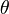$\theta$ is the angle
d$\theta$ and d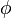$\phi$ are the change in angles

Inputting the equations for escape velocity, time dilation, and length contraction (equations 1, 2, and 3) into the equation for flat spacetime (equation 4), to get the Schwarzschild metric: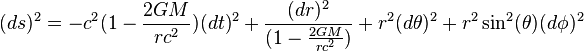$(ds)^{2}=-c^{2}(1-\frac{2GM}{rc^{2}})(dt)^{2}+\frac{(dr)^{2}}{(1-\frac{2GM}{rc^{2}})}+r^{2}(d\theta)^{2}+r^{2}\sin^{2}(\theta)(d\phi)^{2}$ (5)

From this equation we can take out the Schwarzschild radius (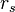$r_{s}$), the radius of this black hole. Although this is most commonly used to describe a Schwarzschild black hole, the Schwarzschild radius can be calculated for any heavy object.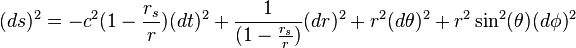$(ds)^{2}=-c^{2}(1-{\frac {r_{s}}{r}})(dt)^{2}+{\frac {1}{(1-{\frac {r_{s}}{r}})}}(dr)^{2}+r^{2}(d\theta )^{2}+r^{2}\sin ^{2}(\theta )(d\phi )^{2}$ (6)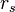$r_s$ is the set radius limit of the object

## Images for kidsSchwarzschild metric Facts for Kids. Kiddle Encyclopedia.